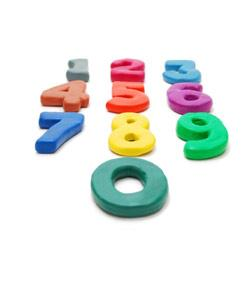# Ub04 - Fl 4 - Digit 1 - Type Of Facility

7 Questions | Total Attempts: 110SettingsQuiz over first digit of type of bill field locator on UB 04.

• 1.
If the first digit is 1 then the type of facility is a:
• 2.
If the first digit is a 2 then the type of facility is a:
• 3.
If the first digit is a 3 then the facility type is a:
• 4.
If the the first digit is a 4 then the type of facility is a:
• 5.
If the first digit is a 5 then the type of facility:
• 6.
If the first digit is a 7 then the type of facility is a:
• 7.
If the first digit is 8 then the type of facility is a:
Related TopicsBack to top